Geosci. Model Dev., 15, 5407–5419, 2022
https://doi.org/10.5194/gmd-15-5407-2022
Geosci. Model Dev., 15, 5407–5419, 2022
https://doi.org/10.5194/gmd-15-5407-2022
Development and technical paper | 15 Jul 2022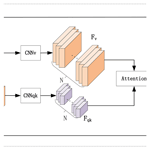# RAP-Net: Region Attention Predictive Network for precipitation nowcasting

RAP-Net: Region Attention Predictive Network for precipitation nowcasting
Zheng Zhang1,, Chuyao Luo1,, Shanshan Feng1, Rui Ye1, Yunming Ye1, and Xutao Li1 Zheng Zhang et al.
• 1School of Computer Science and Technology, Harbin Institute of Technology, Shenzhen, China
• These authors contributed equally to this work.

Correspondence: Xutao Li (lixutao@hit.edu.cn)

Abstract

Natural disasters caused by heavy rainfall often cause huge loss of life and property. Hence, the task of precipitation nowcasting is of great importance. To solve this problem, several deep learning methods have been proposed to forecast future radar echo images, and then the predicted maps are converted to the distribution of rainfall. The prevailing spatiotemporal sequence prediction methods apply a ConvRNN structure, which combines the convolution and recurrent neural network. Although ConvRNN methods achieve remarkable success, they do not capture both local and global spatial features simultaneously, which degrades the nowcasting in regions of heavy rainfall. To address this issue, we propose a Region Attention Block (RAB) and embed it into ConvRNN to enhance forecasting in the areas with heavy rainfall. Besides, the ConvRNN models find it hard to memorize longer historical representations with limited parameters. To this end, we propose a Recall Attention Mechanism (RAM) to improve the prediction. By preserving longer temporal information, RAM contributes to the forecasting, especially in the moderate rainfall intensity. The experiments show that the proposed model, Region Attention Predictive Network (RAP-Net), significantly outperforms state-of-the-art methods.

Share
1 Introduction

Precipitation nowcasting has vital influence in the fields of transportation, agriculture, tourism, industry, and city alarming. Due to the higher spatial and temporal resolution of the radar echo image, it is effective for forecasting the distribution of rainfall by predicting the future radar echo maps and converting each pixel to the rainfall intensity according to the ZR relationship . Therefore, precipitation nowcasting is often defined as a spatiotemporal prediction problem.

Traditional approaches to precipitation nowcasting are motion-field-based methods. The specific process can be briefly divided into three parts. First, the motion field is estimated by variational radar echo-tracking methods such as optical flow . Second, the future radar reflectivities are advected by a semi-Lagrangian advection scheme under the assumption of stationary movement. Third, the performance of the forecasts is evaluated by comparing them to ground truth. However, these methods do not exploit abundant historical observations.

To overcome this limitation, some deep learning-based methods have been proposed to handle precipitation nowcasting . They usually build a mapping from previous observations to future maps by learning from the abundant historical radar data. Generally, the prevailing approaches utilize the structure of ConvRNN, which combines the convolution neural network (CNN) and recurrent neural network (RNN). Furthermore, to enhance the spatiotemporal representation ability, other types of neural networks such as the spatial transformer network (STN; Shi et al.2017), deformable convolution network (DCN; Wu et al.2021), and attention module are introduced in the ConvRNN unit and obtain better performance.

However, existing ConvRNN models confront the following three drawbacks: (1) the convolution employed in the current input only extracts the local features instead of the large-scale representation due to a fixed kernel size. It may lead to useful information beyond the visual field of convolution not being captured, and this thus degrades the performance. (2) The convolution applied in the previous hidden states only transmits local previous representations to the current states, which means historical spatial information cannot be fully used. (3) The update process of the temporal memory limits the long-term spatiotemporal representation preservation. Thus, the information including high echo reflectivity is easily dropped. Although some remedial solutions based on the attention mechanism are proposed, they are hard to apply in large-scale inputs and long-term predictions due to the limitation of space occupation.

To address the first two problems, we propose a Region Attention Block (RAB) and embed it into the input and hidden state, respectively. It simultaneously exploits the global spatial representation and preserves the local feature. RAB classifies each feature map into equal-sized tensors, and each tensor gatherers a similar semantic. Then, the attention module is used to interact with the contents of all semantics. To this end, the large-scale feature map can be captured from the global view and, meanwhile, maintain local representations. Therefore, the large-scale spatial feature of the current input and previous hidden states can be preserved. Moreover, to capture the long-term spatiotemporal dependency of representation without increasing parameters, we present the Recall Attention Mechanism (RAM) to retrieval all historical inputs. More rainfall information is captured by this component. By combining these modules, the performance for heavy and moderate rainfall can be significantly improved. In brief, the main contributions of the paper are summarized as follows:

1. We first propose a new attention method, namely Region Attention Block (RAB), to capture both global and local spatial features simultaneously to improve the spatial expressivity of feature maps.

2. We embed the RAB into current inputs and previous hidden states to obtain the large-scale spatial information from the global view and persevere different semantics at the same time. For the same echo with a large-scale size and long-range movement between the adjacent time, more useful spatial information can be extracted, which leads to more accurate predictions in those regions with heavy rainfall.

3. We propose the Recall Attention Mechanism (RAM) to retrieval all historical inputs with limited parameters. The representation of moderate and heavy rainfall intensity can be preserved in the predicted unit.

2 Related work

Traditional methods mainly focus on estimating the motion field between the adjacent radar maps, and then the next prediction can be extrapolated based on this movement. Here, the motion field describes the direction and distance of each pixel that needs to be moved at the next moment. To obtain the movement, tracking radar echoes by correlation (TREC; Wang et al.2013) divides the whole radar map into serval equal-sized boxes and calculates the motion vector of each pair's box center by searching the highest correlation between boxes at the adjacent time steps. Another type of approach is the optical flow-based method . It calculates the motion field under a pixel level, based on the assumption that the brightness of pixels remains unchanged. Upon the idea, many algorithms are developed to apply the radar maps with the large movement vector. However, the invariant brightness assumption conflicts with the realistic movements of hydrometeors, and massive historical data are utilized.

To overcome it, many deep learning-based methods are proposed to predict the radar sequence without the above unreasonable assumption. Most of the methods commonly exploit the structure of ConvRNN. It combines convolution neural network (CNN) and recurrent neural network (RNN) to preserve the spatiotemporal feature of the historical sequence. Furthermore, Wang et al. added a spatial memory in a predicted unit and an attention mechanism in temporal memory to enhance the spatiotemporal representation ability of the short term and long term, respectively. Although these methods have a remarkable performance, the visualization of their predictions is usually blurry due to the loss function and the architecture of the model (Shouno2020). To handle the issue, a generative adversarial network has been introduced in the ConvRNN model to improve predictive clarity. Nevertheless, the non-convergence and collapse problem would cause a negative influence on the prediction. Our proposed method is different from existing deep learning methods in two aspects. In the short term, the proposed RAB can simultaneously exploit local and global spatiotemporal representations. In the long term, the RAM can effectively recall all historical observations with limited space occupancy.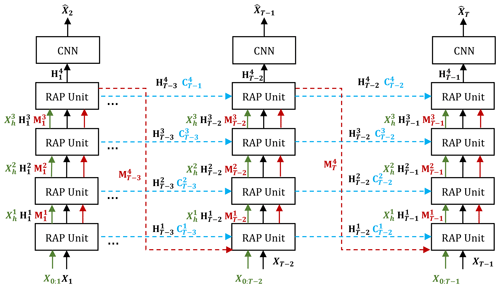Figure 1The overall architecture of the Region Attention Predictive Network (RAP-Net).

3 Proposed method

## 3.1 Problem definition

The precipitation nowcasting task can be defined as the spatiotemporal sequence prediction problem . Based on historical observations X0:t, it aims to forecast the future radar echo images ${\stackrel{\mathrm{‾}}{X}}_{t+\mathrm{1}:T}$ that have maximum probability with ground truth ${X}_{t+\mathrm{1}:T}$, as follows:

$\begin{array}{}\text{(1)}& {\stackrel{\mathrm{‾}}{X}}_{t+\mathrm{1}:T}=\mathrm{arg}maxP\left({X}_{t+\mathrm{1}:T}|{X}_{\mathrm{0}},{X}_{\mathrm{1}},\mathrm{\dots },{X}_{t}\right).\end{array}$

In this paper, t and T are set to 5 and 15, respectively, which means that 10 continuous radar maps need to be predicted according to five historical images.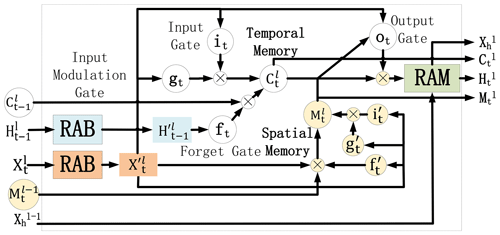Figure 2The internal structure of the Region Attention Predictive Unit (RAP-Unit).

## 3.2 Overall architecture

The overall architecture of the proposed model RAP-Net is presented in Fig. 1. It utilizes the structure of PredRNN and stacks several RAP-Units to generate the predictions from timestamp 2 to T. At any timestamp t, the model predicts a radar map ${\stackrel{\mathrm{^}}{X}}_{t+\mathrm{1}}$ at the next timestamp t+1, according to the current radar map Xt and historical radar sequence X0:t. The red and blue arrows denote the delivery direction of the spatial memory M and temporal memory C, respectively. These two memories preserve spatial and temporal representations, respectively. Different from the PredRNN, RAP-Net exploits dissimilar data flow to transmit long-term spatiotemporal information ${X}_{h}^{l}$ which preserves all historical representations. Besides, we notice that the majority of ConvRNN models employ similar architecture. Hence, the difference lies in their units instead of the employed architecture. In the experiment section, we will discuss and analyze the performance of different units utilized by the existed methods.

The internal structure of Region Attention Predictive Unit (RAP-Unit) is shown in Fig. 2. The inputs include the current input ${X}_{t}^{l}$, previous hidden state ${H}_{t-\mathrm{1}}^{l}$, temporal memory ${C}_{t-\mathrm{1}}^{l}$, spatial memory ${M}_{t}^{l-\mathrm{1}}$, and long-term historical representation ${X}_{h}^{l-\mathrm{1}}$. According to Fig. 1, RAP-Net consists of four stacked RAP-Units. At the bottom layer, ${X}_{h}^{l-\mathrm{1}}$ represents all historical inputs X0:t, while at other layers, ${X}_{h}^{l-\mathrm{1}}$ is the output of the previous layer. The outputs of RAP-Unit are the current hidden state ${H}_{t}^{l}$, spatial memory ${M}_{t}^{l}$, temporal memory ${C}_{t}^{l}$, and new long-term representation ${X}_{h}^{l}$. The details of the calculation are presented according to the following formulas:

$\begin{array}{}\text{(2)}& \begin{array}{rl}& {{X}^{\prime }}_{t}^{l}=\mathrm{RAB}\left({X}_{t}^{l}\right),\\ & {{H}^{\prime }}_{t-\mathrm{1}}^{l}=\mathrm{RAB}\left({H}_{t-\mathrm{1}}^{l}\right),\\ & {i}_{t}=\mathit{\sigma }\left({W}_{xi}*{{X}^{\prime }}_{t}^{l}+{W}_{hi}*{{H}^{\prime }}_{t-\mathrm{1}}^{l}+{b}_{i}\right),\\ & {g}_{t}=\mathrm{tanh}\left({W}_{xg}*{{X}^{\prime }}_{t}^{l}+{W}_{hg}*{{H}^{\prime }}_{t-\mathrm{1}}^{l}+{b}_{g}\right),\\ & {f}_{t}=\mathit{\sigma }\left({W}_{xf}*{{X}^{\prime }}_{t}^{l}+{W}_{hf}*{{H}^{\prime }}_{t-\mathrm{1}}^{l}+{b}_{f}\right),\\ & {i}_{t}^{\prime }=\mathit{\sigma }\left({W}_{xi}^{\prime }*{{X}^{\prime }}_{t}^{l}+{W}_{mi}*{M}_{t}^{l-\mathrm{1}}+{b}_{i}^{\prime }\right),\\ & {g}_{t}^{\prime }=\mathrm{tanh}\left({W}_{xg}^{\prime }*{{X}^{\prime }}_{t}^{l}+{W}_{mg}*{M}_{t}^{l-\mathrm{1}}+{b}_{g}^{\prime }\right),\\ & {f}_{t}^{\prime }=\mathit{\sigma }\left({W}_{xf}^{\prime }*{{X}^{\prime }}_{t}^{l}+{W}_{mf}*{M}_{t}^{l-\mathrm{1}}+{b}_{f}^{\prime }\right),\\ & {C}_{t}^{l}={i}_{t}\circ {g}_{t}+{f}_{t}\circ {C}_{t-\mathrm{1}}^{l},\\ & {M}_{t}^{l}={i}_{t}^{\prime }\circ {g}_{t}^{\prime }+{f}_{t}^{\prime }\circ {M}_{t}^{l-\mathrm{1}},\\ & {o}_{t}=\mathit{\sigma }\left({W}_{xo}*{{X}^{\prime }}_{t}^{l}+{W}_{ho}*{{H}^{\prime }}_{t-\mathrm{1}}^{l}+{W}_{co}*{C}_{t}^{l}+\\ & \phantom{\rule{1em}{0ex}}{W}_{mo}*{M}_{t}^{l}+{b}_{o}\right),\\ & {H}_{t}^{l}={o}_{t}\circ \mathrm{tanh}\left({W}_{\mathrm{1}×\mathrm{1}}*\left[{{X}^{\prime }}_{t}^{l},{M}_{t}^{k}\right]\right),\\ & {H}_{t}^{l},{X}_{h}^{l}=\mathrm{RAM}\left({H}_{t}^{l},{X}_{h}^{l-\mathrm{1}}*{W}_{l}\right),\end{array}\end{array}$

where the * and symbols denote the convolution and Hadamard product, respectively. it, gt, ft, ${i}_{t}^{\prime }$, ${g}_{t}^{\prime }$, and ${f}_{t}^{\prime }$ indicate various gates, which can be viewed as intermediate variables. Here, RAB and RAM are the Region Attention Block and Recall Attention Mechanism, respectively.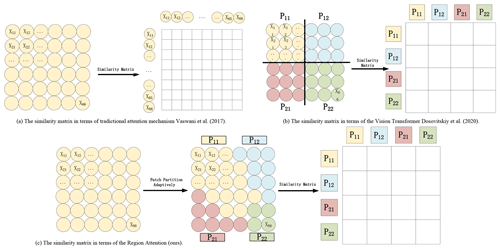Figure 3The calculation process of a similarity matrix, based on three different attention methods.​​​​​​​

## 3.3 Region Attention Block

To address the issue, we expect that these patches can be divided adaptively, and those elements with similar semantic relationships are classified into the same patch, as shown in Fig. 3c​​​​​​​. To realize this idea, we propose the Region Attention Block (RAB), whose structure is illustrated in Fig. 4. First, a convolution and softmax layer are employed in the input feature map ${F}^{i}\in {R}^{B×C×H×W}$ to generate ${F}^{c}\in {R}^{B×N×H×W}$ to distinguish the N classifications. Second, the original input Fi is split into N groups of feature maps ${F}^{n}\in {R}^{N×B×C×H×W}$ by the split module, following this formula:

$\begin{array}{}\text{(3)}& \begin{array}{rl}\mathrm{Split}\left({F}^{i},{F}^{c}\right)& =\mathrm{Concatenate}\left(\mathit{\left\{}{F}_{j,k,h,w}^{i}\cdot {F}_{j,n,h,w}^{c}|\mathrm{1}

These groups denote various semantic information extracted from different positions. Third, ${F}^{qk}\in {R}^{B×N×c×h×w}$ is convolved by Fn to further exploit the feature of Fn and reduce the parameters, where the c, h, and w are smaller than C, H, and W. Besides, ${F}^{v}\in {R}^{N×B×C×H×W}$ are outputted by a convolution layer applied in Fn to preserve the original information. Fourth, three different convolutions are used to generate query Qs, key Ks, and value Vs, based on Fqk and Fv. After flattening, ${Q}_{s}\in {R}^{B×N×c*h*w}$, ${K}_{s}\in {R}^{B×N×c*h*w}$ and ${V}_{s}\in {R}^{B×N×C*H*W}$ are fed into the spatial attention function to obtain ${F}^{a}\in {R}^{B×N×C×H×W}$ that have been interacting with the local representation from different regions. The output after the attention function is as follows:

$\begin{array}{}\text{(4)}& \mathrm{Attention}\left({Q}_{s},{K}_{s},{V}_{s}\right)=\mathrm{softmax}\left(\frac{f\left({Q}_{s},{K}_{s}^{T}\right)}{\sqrt{{d}_{k}}}\right){V}_{s},\end{array}$

where f denotes the dot product, and dk is the dimension of key Ks. $f\left({Q}_{s},{K}_{s}^{T}\right)\in {R}^{B×N×N}$ is the similarity matrix of various semantics in different regions. Fifth, an integration module is utilized to integrate Fa based on the Fc and obtains the result ${F}^{{a}^{\prime }}$ from this equation, as follows:

$\begin{array}{}\text{(5)}& \begin{array}{rl}{F}^{{a}^{\prime }}& =\mathrm{Integration}\left({F}^{a},{F}^{c}\right)\\ & =\sum _{n=\mathrm{1}}^{N}{F}_{j,k,h,w}^{a}\cdot {F}_{j,n,k,h,w}^{c},\end{array}\end{array}$

where, ${F}^{{a}^{\prime }}$ has the same size as input feature map Fi. Finally, the structure of ResNet is introduced to deeply exploit the spatial feature and achieve the final result ${F}^{o}\in {R}^{B×N×C×H×W}$. In summary, the calculation process is described by the following formulas:

$\begin{array}{}\text{(6)}& \begin{array}{rl}& {F}^{c}=\mathrm{softmax}\left({F}^{i}*{W}_{c}\right),\\ & {F}^{n}=\mathrm{Split}\left({F}^{i},{F}^{c}\right),\\ & {F}^{qk}={F}^{n}*{W}_{qk}^{\prime },\\ & {F}^{v}={F}^{n}*{W}_{v}^{\prime },\\ & {F}^{a}=\mathrm{Attention}\left({F}^{qk}*{W}_{q},{F}^{qk}*{W}_{k},{F}^{v}*{W}_{v}\right),\\ & {F}^{{a}^{\prime }}=\mathrm{Integration}\left({F}^{a},{F}^{c}\right),\\ & {F}^{o}={F}^{i}+{F}^{{a}^{\prime }}.\end{array}\end{array}$

The traditional attention mechanism calculates, in Fig. 3a, the similarity between different pixels, and the attention manner of the vision transformer, in Fig. 3b, compares different regions in a fixed location. Different from both mechanisms, the attention similarity from region attention (ours), in Fig. 3c, compares the difference between regions with a flexible size and position. Due to the irregular shape of radar echo and the different distributions, RAB can capture the correlation between the different radar echoes better. Therefore, the introduction of this block can improve the spatiotemporal ability of the model, especially since the information of radar echoes with high reflectivity is more easily extracted because they have a more stable appearance and shape. RAM has more of a contribution to improving the performance in these regions with heavy rainfall.

## 3.4 Recall Attention Mechanism

To capture the temporal long dependencies of representation, embedded the spatial attention module in the updating of temporal memory. However, it has the following two limitations: (1) it saves abundant history temporal memories, which leads to the number of parameters easily exceeding the space occupancy as the lead time goes. (2) The temporal memory has lost some information during the generation of various gates. Therefore, the preserved previous representation fails to capture all the information, and long-term spatiotemporal expressivity is limited.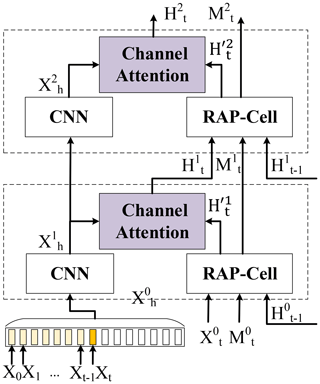Figure 5The manner of embedding the Recall Attention Mechanism (RAM) into the proposed predicted unit. Here, the RAP-Cell is the RAP unit without RAM.

Table 1The parameter settings of the RAP-Unit. The term “In res” and “Out res” denote the resolutions of input and output, respectively, while “Conv” is the convolution operation.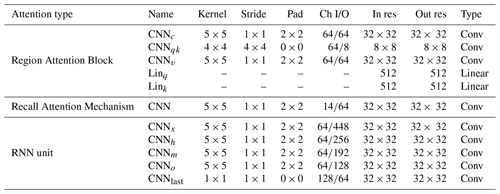To address these issues, we propose the Recall Attention Mechanism (RAM) to enhance the long-term spatiotemporal representation ability with a fixed space occupation, as Fig. 5 shows. First, we build an empty long-memory feature map ${X}_{h}^{\mathrm{0}}\in {R}^{B×T×C×H×W}$ in the bottom layer and feed the current input Xt into it continually. Note that the ${X}_{h}^{\mathrm{0}}$ contains all original previous inputs ${X}_{\mathrm{0}:t}\in {R}^{B×T×C×H×W}$. Second, a convolution neural network is employed to extract the feature of ${X}_{h}^{\mathrm{0}}$ and output the long-memory hidden state ${X}_{h}^{\mathrm{1}}\in {R}^{B×T×C×H×W}$. Last, ${X}_{h}^{\mathrm{1}}$ and the output ${{H}^{\prime }}_{t}^{\mathrm{1}}$ of the RAP-Cell (which can be regarded as the RAP-Net model without RAM) feed into the channel attention module to generate new hidden states, where the ${X}_{h}^{\mathrm{1}}$ can be regarded as the key ${K}_{c}\in {R}^{B×T*C×H*W}$ and value ${V}_{c}\in {R}^{B×T*C×H*W}$, and the ${H}_{t}^{\prime }$ represents the query ${Q}_{c}\in {R}^{B×C×H*W}$. The formula of channel attention is shown as follows:

$\begin{array}{}\text{(7)}& \mathrm{Attention}\left({Q}_{c},{K}_{c},{V}_{c}\right)=\mathrm{softmax}\left(\frac{f\left({Q}_{c},{K}_{c}^{T}\right)}{\sqrt{{d}_{k}}}\right){V}_{c},\end{array}$

where the f denotes the dot product, and dk is the dimension of key Kc. $f\left({Q}_{c},{K}_{c}^{T}\right)\in {R}^{B×T×T*C}$ is the similarity matrix between channels of Qs and channels of Kc. From Eq. (7), we can see that the Vc can be extracted according to the $f\left({Q}_{c},{K}_{c}^{T}\right)$, where Qc decides how to explore the Vc by dot-producing with Kc. Therefore, the original output ${{H}^{\prime }}_{t}^{\mathrm{1}}$ of the RAP-Cell can be regarded as query Qc to explore long-term spatiotemporal representations ${X}_{h}^{\mathrm{1}}$ that are the key Kc and value Vc. In this way, the new output ${H}_{t}^{\mathrm{1}}$ has recalled all original historical representations, and long-term dependencies can be effectively preserved. Besides, the size of the long-memory feature map ${X}_{h}^{l}$ is fixed at any time step because the size of Xh is predefined, and the corresponding content at different timestamps are fed into Xh. Similarly, in the lth layer, the input of the long-memory hidden state is the ${X}_{h}^{\mathrm{1}}$. In the bottom layer, ${X}_{h}^{l}$ is the result after convolution by historical input sequences X0:t. In the other layers, the ${X}_{h}^{l}$ is the result after convolution by the ${X}_{h}^{l-\mathrm{1}}$. By RAM, the long-term historical representation can be delivered to the next layer.

4 Experiments

## 4.1 Dataset

The dataset is collected from the CIKM AnalytiCup2017 competition (available at https://tianchi.aliyun.com/competition/entrance/231596/information, ), which covers the whole area of Shenzhen, China. For convenience, we name this public dataset RadarCIKM. RadarCIKM has a training set and test set with 10 000 and 4000 sequences, respectively. There are 2000 sequences randomly sampled from the training set to build the validation set. Each sequence contains 15 continual observations within 90 min, where the spatial and temporal resolution of each map is 101 × 101 and 6 min, respectively. The range of each pixel is from 0 to 255, and each pixel denotes 1 km × 1 km. Moreover, the type of pixel is an integer, and each value can be converted to radar reflectivity (dBZ) by the following equation:

$\begin{array}{}\text{(8)}& \mathrm{dBZ}=p×\frac{\mathrm{95}}{\mathrm{255}}-\mathrm{10}.\end{array}$

Then, the rainfall intensity can be obtained by the radar reflectivity (dBZ) and ZR relationship as follows:

$\begin{array}{}\text{(9)}& \mathrm{dBZ}=\mathrm{10}\mathrm{log}a+\mathrm{10}b\mathrm{log}R,\end{array}$

where the R is the rain rate level, a=58.53, and b=1.56.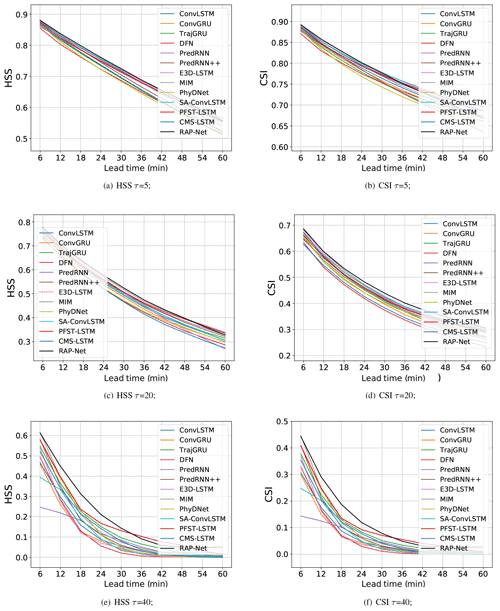Figure 6The HSS and CSI scores of different case lead time values (best viewed in color).​​​​​​​

## 4.2 Evaluation metrics

In this paper, in addition to common measurements such as structural similarity (SSIM) and mean absolute error (MAE) in video prediction, we also utilize the Heidke skill score (HSS) and critical success index (CSI) that are commonly used in precipitation nowcasting tasks. The HSS evaluates the fraction of correct forecasts after eliminating random predictions. The CSI measures the number of correct forecasts divided by the total number of occasions when the rainfall events were forecasted or observed. Specifically, the prediction and ground truth are converted to a binary matric based on a threshold τ. When the value of the dBZ is larger than τ, then it is set to 1 or otherwise to 0. Next, the number of the true positive (TP; prediction = 1; truth = 1), false negative (FN; prediction = 0; truth = 1), false positive (FP; prediction = 1; truth = 0), and true negative (TN; prediction = 0; truth = 0) are counted. Finally, the HSS and CSI can be calculated by the following formulas:

$\begin{array}{}\text{(10)}& \mathrm{HSS}=\frac{\mathrm{2}\left(\mathrm{TP}×\mathrm{TN}-\mathrm{FN}×\mathrm{FP}\right)}{\left(\mathrm{TP}+\mathrm{FN}\right)\left(\mathrm{FN}+\mathrm{TN}\right)+\left(\mathrm{TP}+\mathrm{FP}\right)\left(\mathrm{FP}+\mathrm{TN}\right)},\text{(11)}& \mathrm{CSI}=\frac{\mathrm{TP}}{\mathrm{TP}+\mathrm{FN}+\mathrm{FP}}.\end{array}$

Here, the range of HSS, CSI, and SSIM is [0,1]. The range of MAE is [$\mathrm{0},+\mathrm{\infty }$].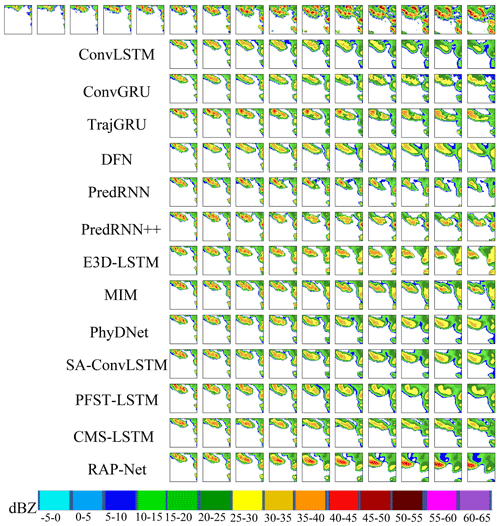Figure 7The first row is the reflectivity of the ground truth, and the remaining rows show the predicted reflectivity of various methods on an example from the RadarCIKM dataset (best viewed in color).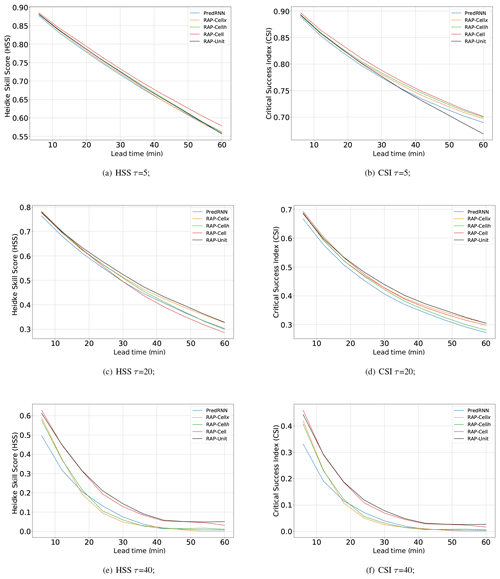Figure 8The performance changes against different nowcast lead times in terms of HSS and CSI scores in the ablation study (best viewed in color).

## 4.3 Parameters setting

The proposed RAP-Net takes five previous radar echo maps as inputs and outputs 10 predictions. It utilizes four layers of RAP-Units, as shown in Fig. 1, and the parameters setting of each RAP-Unit are shown in Table 1. The Adam optimizer is applied to train our model with a learning rate of 0.0004. Besides, the early stopping and scheduled sampling strategies are also used to optimize our model. The loss function combines the L1 and L2 to train RAP-Net. All experiments are implemented in Pytorch and conducted on NVIDIA 3090 graphics processing units (GPUs).

## 4.4 Result and analysis

Table 2 shows the results of all models. The best results are in boldface and the second best scores are underlined. We find that the RAP-Net achieves the smallest error and the highest structural similarity according to the MAE and SSIM. It is observed that our model outperforms other models in terms of the comprehensive performance. Besides, the proposed model has significant superiority especially for the nowcasting in heavy rainfall regions. Because the HSS and CSI keep the top position in the middle and high thresholds (20 and 40 dBZ). For the state-of-art method, PFST-LSTM , all measurements of it are exceeded by RAP-Net, which shows the performance of our model. Comparing with PredRNN, PredRNN++, and RAP-Net, we can see that they have a similar SSIM due to applying the same architecture. However, the other evaluation scores of RAP-Net are significantly higher than PredRNN and PredRNN++, which implies the benefit of RAP-Unit. Last, we notice that the SA-ConvLSTM has the best HSS and CSI in the lowest threshold (5 dBZ). Nevertheless, its performance is poor in the highest threshold (40 dBZ), which implies that the RAB and RAM can improve the prediction in the area with a high radar echo compared to the traditional attention mechanism because the main difference between the RAP-Net and SA-ConvLSTM is that they introduce different attention submodules.

Table 2Comparison results on RadarCIKM in terms of HSS, CSI, SSIM, and MAE. The best results are given in bold, and the second-best scores are underlined.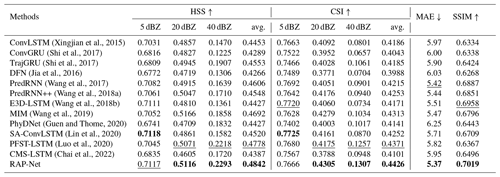Figure 9The first row is the reflectivity of the ground truth and the remaining rows are the predicted reflectivity of different methods on an example from the RadarCIKM dataset (best viewed in color).

To show the performances of various models at different nowcasting lead times, Fig. 6 presents the HSS and CSI curves with regard to different lead times under all thresholds. We observe that both HSS and CSI scores of all models decrease as the lead time increases, which shows the difficulty of long-term predictions. Among these models, RAP-Net achieves notable superiority in the middle and late stages of the nowcasting period at the highest threshold. Especially in the last prediction, all baseline methods trend to obtain the same poor result. The RAP-Net remarkably outperforms other models. It implies that the introduction of RAB and RAM in the proposed model contributes to generating long-term predictions within heavy rainfall regions. Although the performance of RAP-Net would be degraded when the threshold becomes small, it still has competitiveness compared to other models.

Figure 7 shows an example of predictions from these models. The various colors denote the different ranges of reflectivity according to the color bar in the bottom of Fig. 7. From the ground truth in the first row, the rainfall event is obviously the trend of increasing the rainfall intensity. However, only our model can forecast this trend and keep the intensity of the regions. The RAP-Net can generate a high reflectivity area, which can also explain why our model can achieve the highest evaluation index HSS and CSI in the middle and high thresholds.

Table 3Ablation results on RadarCIKM in terms of HSS, CSI, MAE, and SSIM. The best results are given in bold, and the second-best scores are underlined.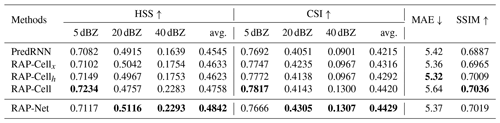## 4.5 Ablation study

To investigate the influence of various modules, we conduct an ablation study to discuss the effectiveness of Region Attention Block to the current input and the last hidden state. The result of evaluations is shown in Table 3. RAP-Cellx and RAP-Cellh denote the PredRNN model embedding the RAB into the input and hidden state, respectively. The RAP-Cell model is the combination of RAP-Cellx and RAP-Cellh and can also be regarded as RAP-Net without RAM. The results of RAP-Cellx and RAP-Cellh are higher than PredRNN, which shows the advantage of introducing the Region Attention Block, especially since RAP-Cellh significantly reduces the error according to MAE. Besides, the HSS, CSI, and SSIM of the RAP-Cell have significant improvements, particularly when the threshold τ is 40 dBZ, which implies that RAB being simultaneously employed in the input and hidden state contributes to the prediction in the heavy rainfall regions. Moreover, by comparing the RAP-Cell and RAP-Net, we find that the RAM can enhance the accuracy of the nowcasting, especially in the areas with moderate intensity rainfall.

Similarly, we also plot Fig. 8 to show the experimental results of all models against different nowcast lead times. We can see that RAP-Net delivers more promising results when the threshold increases, which demonstrates the effectiveness of combining RAB and RAM in terms of long-term prediction in a high reflectivity area. The performance of RAM can be shown by comparing RAP-Cell and RAP-Net. We notice that the introduction of RAM can improve the prediction in the regions of moderate rainfall intensity. Besides, RAP-Cellx and RAP-Cellh embed RAB in the current input and the hidden state, respectively. Their performance is better than the original model PredRNN, especially in the 20 dBZ threshold. It shows the superiority of RAB.

We also show predictions of different methods for a given sample in Fig. 9. We find that RAP-Cell can generate the red area which is reflected by better evaluation indexes of HSS and CSI in the highest threshold. However, all forecasts, except for RAP-Net, have a gap in the radar echo block, which is obviously different from the ground truth. The improvement of prediction in moderate rainfall intensity regions can be owed to the embedding of RAM.

5 Conclusions

In this paper, we propose the RAP-Net to handle the precipitation nowcasting task. On the one hand, it embeds the Region Attention Block to enhance the local and global spatial representation ability simultaneously by extracting and delivering the features in ConvRNN. The improvement can significantly enhance the accuracy, especially in those regions with heavy rainfall. On the other hand, we introduce the Recall Attention Mechanism to improve the temporal expressivity in the long term. It can preserve and retrieve longer historical information and effectively enhance the performance of prediction, particularly for the moderate rainfall intensity regions. We conduct extensive experiments to evaluate the performances of most ConvRNN models. Empirically, RAP-Net can preserve regions of heavy intensity in long-term predictions. It shows the effectiveness of RAB and RAM in improving forecasting. The ablation study independently measures the influence of these two modules. The RAB is able to enhance the accuracy in the high threshold, and RAM can improve the prediction in the middle threshold.

Currently, most of the existing methods focus on radar echo maps prediction based on a single altitude layer. The variety and movement of the echo not only need to consider the previous sequence in the same layers but also need to use different altitude layers because the hydrometeors not only happen in the horizontal direction but also act in the vertical direction. For future work, we will consider integrating other layers' historical information to improve the forecasting. In detail, we intend to utilize channel attention to exploit the spatiotemporal representations and then integrate those into the RAP unit. After training, the model can adaptively extract valid spatial information from different levels. We will perform further experiments on multi-channel RAP-Net based on multi-layers of radar echo images. Besides, by a visualization of the similarity matrix in channel attention, the level which is more important for final predictions can be found out.

Code availability

The source code and pretrained model of RAP-Net are available at https://doi.org/10.5281/zenodo.5979275 .

Data availability

The data are available at https://doi.org/10.5281/zenodo.5979275 .

Author contributions

Conceptualization, methodology, and writing of the investigation were performed by ZZ and CL. RY contributed to the project administration and visualization. SF and XL contributed to writing, review, editing, data curation, and validation. YY contributed to supervision, visualization, investigation, resources, and funding acquisition.

Competing interests

The contact author has declared that none of the authors has any competing interests.

Disclaimer

Publisher’s note: Copernicus Publications remains neutral with regard to jurisdictional claims in published maps and institutional affiliations.

Acknowledgements

This work has been supported in part by the Shenzhen Science and Technology Program, China (grant nos. JCYJ20200109113014456 and JCYJ20210324120208022).

Financial support

This work has been supported in part by the Shenzhen Science and Technology Program (grant nos. JCYJ20180507183823045, JCYJ20200109113014456, and JCYJ20210324120208022).

Review statement

This paper was edited by Charles Onyutha and reviewed by three anonymous referees.

References

Alibaba Cloud: CIKM AnalytiCup2017 competition, Alibaba Cloud [data set], https://tianchi.aliyun.com/competition/entrance/231596/information, last access: 2022. a

Ayzel, G., Scheffer, T., and Heistermann, M.: RainNet v1.0: a convolutional neural network for radar-based precipitation nowcasting, Geosci. Model Dev., 13, 2631–2644, https://doi.org/10.5194/gmd-13-2631-2020, 2020. a

Chai, Z., Yuan, C., Lin, Z., and Bai, Y.: CMS-LSTM: Context-Embedding and Multi-Scale Spatiotemporal-Expression LSTM for Video Prediction, arXiv [preprint], https://doi.org/10.48550/arXiv.2102.03586, April 2022. a

Fu, J., Liu, J., Tian, H., Li, Y., Bao, Y., Fang, Z., and Lu, H.: Dual attention network for scene segmentation, in: CVPR, pp. 3146–3154, https://doi.org/10.1109/CVPR.2019.00326, 2019. a

Guen, V. L. and Thome, N.: Disentangling physical dynamics from unknown factors for unsupervised video prediction, in: CVPR, pp. 11474–11484, https://doi.org/10.1109/CVPR42600.2020.01149, 2020.​​​​​​​ a

He, K., Zhang, X., Ren, S., and Sun, J.: Deep residual learning for image recognition, in: CVPR, pp. 770–778, https://doi.org/10.1109/CVPR.2016.90, 2016. a

Jia, X., De Brabandere, B., Tuytelaars, T., and Gool, L. V.: Dynamic filter networks, NIPS, 29, 667–675, 2016. a

Li, D., Liu, Y., and Chen, C.: MSDM v1.0: A machine learning model for precipitation nowcasting over eastern China using multisource data, Geosci. Model Dev., 14, 4019–4034, https://doi.org/10.5194/gmd-14-4019-2021, 2021. a

Lin, Z., Li, M., Zheng, Z., Cheng, Y., and Yuan, C.: Self-attention convlstm for spatiotemporal prediction, in: AAAI, vol. 34, pp. 11531–11538, https://ojs.aaai.org/index.php/AAAI/article/view/6819 (last access: July 2022), 2020. a, b, c, d

Luo, C., Li, X., and Ye, Y.: PFST-LSTM: A SpatioTemporal LSTM Model With Pseudoflow Prediction for Precipitation Nowcasting, IEEE J. Sel. Top. Appl., 14, 843–857, 2020. a, b

Luo, C., Li, X., Wen, Y., Ye, Y., and Zhang, X.: A Novel LSTM Model with Interaction Dual Attention for Radar Echo Extrapolation, Remote Sensing, 13, 164, https://doi.org/10.3390/rs13020164, 2021. a

Pulkkinen, S., Nerini, D., Pérez Hortal, A. A., Velasco-Forero, C., Seed, A., Germann, U., and Foresti, L.: Pysteps: an open-source Python library for probabilistic precipitation nowcasting (v1.0), Geosci. Model Dev., 12, 4185–4219, https://doi.org/10.5194/gmd-12-4185-2019, 2019. a

Ryu, S., Lyu, G., Do, Y., and Lee, G.: Improved rainfall nowcasting using Burgers' equation, J. Hydrol., 581, 124140, https://doi.org/10.1016/j.jhydrol.2019.124140​​​​​​​, 2020. a

Shi, X., Gao, Z., Lausen, L., Wang, H., Yeung, D.-Y., Wong, W.-K., and Woo, W.-C.: Deep learning for precipitation nowcasting: A benchmark and a new model, NIPS, https://proceedings.neurips.cc/paper/2017/hash/a6db4ed04f1621a119799fd3d7545d3d-Abstract.html (last access: July 2022), 2017. a, b, c, d, e, f

Shouno, O.: Photo-realistic video prediction on natural videos of largely changing frames, arXiv [preprint], https://doi.org/10.48550/arXiv.2003.08635, 19 March 2020. a

Tian, L., Li, X., Ye, Y., Xie, P., and Li, Y.: A generative adversarial gated recurrent unit model for precipitation nowcasting, IEEE Geosci. Remote S., 17, 601–605, 2019. a

Trebing, K., Staǹczyk, T., and Mehrkanoon, S.: Smaat-unet: Precipitation nowcasting using a small attention-unet architecture, Pattern Recogn. Lett., 145, 178–186, 2021. a

Wang, G., Wong, W., Liu, L., and Wang, H.: Application of multi-scale tracking radar echoes scheme in quantitative precipitation nowcasting, Adv. Atmos. Sci., 30, 448–460, 2013. a

Wang, Y., Long, M., Wang, J., Gao, Z., and Yu, P. S.: Predrnn: Recurrent neural networks for predictive learning using spatiotemporal lstms, in: NIPS, pp. 879–888, https://proceedings.neurips.cc/paper/2017/hash/e5f6ad6ce374177eef023bf5d0c018b6-Abstract.html (last access: July 2022), 2017. a, b, c, d, e

Wang, Y., Gao, Z., Long, M., Wang, J., and Philip, S. Y.: Predrnn++: Towards a resolution of the deep-in-time dilemma in spatiotemporal predictive learning, in: ICML, pp. 5123–5132, PMLR, http://proceedings.mlr.press/v80/wang18b.html (last access: July 2022), 2018aa. a, b, c

Wang, Y., Jiang, L., Yang, M.-H., Li, L.-J., Long, M., and Fei-Fei, L.: Eidetic 3d lstm: A model for video prediction and beyond, in: International conference on learning representations, https://openreview.net/forum?id=B1lKS2AqtX (last access: July 2022), 2018b. a, b, c, d, e, f

Wang, Y., Zhang, J., Zhu, H., Long, M., Wang, J., and Yu, P. S.: Memory in memory: A predictive neural network for learning higher-order non-stationarity from spatiotemporal dynamics, in: CVPR, pp. 9154–9162, https://doi.org/10.1109/CVPR.2019.00937, 2019. a, b, c

Woo, W.-C. and Wong, W.-K.: Operational application of optical flow techniques to radar-based rainfall nowcasting, Atmosphere, 8, 48​​​​​​​, https://doi.org/10.3390/atmos8030048, 2017. a, b

Wu, H., Yao, Z., Wang, J., and Long, M.: MotionRNN: A flexible model for video prediction with spacetime-varying motions, in: CVPR, pp. 15435–15444, https://openaccess.thecvf.com/content/CVPR2021/html/Wu_MotionRNN_A_Flexible_Model_for_Video_Prediction_With_Spacetime-Varying_Motions_CVPR_2021_paper.html (last access: July 2022), 2021. a

Xie, P., Li, X., Ji, X., Chen, X., Chen, Y., Liu, J., and Ye, Y.: An Energy-Based Generative Adversarial Forecaster for Radar Echo Map Extrapolation, IEEE Geosci. Remote S., 1–5, https://doi.org/10.1109/LGRS.2020.3023950, 2020.  a

Xingjian, S., Chen, Z., Wang, H., Yeung, D.-Y., Wong, W.-K., and Woo, W.-C.: Convolutional LSTM network: A machine learning approach for precipitation nowcasting, in: NIPS, pp. 802–810, https://proceedings.neurips.cc/paper/2015/hash/07563a3fe3bbe7e3ba84431ad9d055af-Abstract.html (last access: July 2022), 2015. a

Zhang, Z. and Luo, C.: RAP-Net: Region Attention Predictive Network for Precipitation Nowcasting, Zenodo [code and data set], https://doi.org/10.5281/zenodo.5979275, 2022. a, b

Zheng, K., Liu, Y., Zhang, J., Luo, C., Tang, S., Ruan, H., Tan, Q., Yi, Y., and Ran, X.: GAN–argcPredNet v1.0: a generative adversarial model for radar echo extrapolation based on convolutional recurrent units, Geosci. Model Dev., 15, 1467–1475, https://doi.org/10.5194/gmd-15-1467-2022, 2022. a